# The Rotating-Wave Approximation

The Hamiltonian for a light field interacting with an atomic transition has matrix elements that oscillate at the optical frequency. However, by a suitable change of basis and neglecting terms corresponding to very far-off-resonant optical coupling, the time dependence can often be eliminated from the Hamiltonian, greatly simplifying calculations. In this tutorial, we discuss this approximation, known as the rotating-wave approximation.

As a simple example, we consider two atomic states coupled by a near-resonant monochromatic light field.

 A two-level atomic system.
 The Hamiltonian for this system interacting with a monochromatic optical field with frequency ω and induced Rabi frequency ΩR.

If we write the matrix elements of the light-atom interaction Hamiltonian in terms of exponentials, rather than trigonometric functions, each off-diagonal matrix element is composed of two terms.

 The Hamiltonian expressed in terms of exponential functions.

We can show that, for each of these matrix elements, one of the terms induces a near-resonant coupling, while the other induces a far-off-resonant coupling.

Consider one of the matrix elements written above:
0|H|ω0=-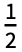ΩR0|- ω t+ ω t|ω0,

whereis the lower state with energy zero, and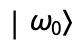is the upper state with energy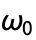. For a near-resonant transition, the energy of the stateshould be close to that of, i.e., zero.

First we consider the term ω t|ω0. Applying the energy operator(with), we have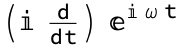|ω0 = -ω ω t|ω0 + ω t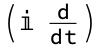|ω0
= -ω ω t|ω0 + ω t(E|ω0)
= -ω ω t|ω0 + ω tω0|ω0
= (ω0-ω) ω t|ω0

In other words, the energy of ω t|ω0 is ω0-ω, which, if ω0ω, is near zero, the energy of the state |0. Thus, if ω0-ωΓ, where Γ is the line width of the upper state, the term 0| ω t|ω0 represents a resonant transition, for which energy is conserved. On the other hand, performing a calculation similar to the above, we find that the energy of - ω t|ω0 is ω0+ω. If ω0ω, then this energy is much greater than the energy of the lower state (ω0+ω>>Γ for optical frequencies). The term 0|- ω t|ω0 represents a far-off-resonant transition. This sort of transition is highly suppressed, and can be neglected.

We can visualize the situation by drawing a level diagram, and indicating the resonant and off-resonant couplings.

 Level diagram for the system, showing both resonant and off-resonant couplings.

One arrow, pointing up from the lower level, nearly matches the separation between the lower and upper states, and represents an optical field detuned by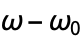from resonance. The other arrow points down from the lower level, and couples the lower level to a point far below resonance (by an amount) with the excited state.

In order to see how we might remove the optical frequencies from the Hamiltonian, we first consider a physically analogous situation that of a spin 1/2 atomic system subject to an oscillating magnetic field.

 Define a second system consisting of the Zeeman sublevels of a spin-1/2 state.

The Hamiltonian for this system subject to an oscillating magnetic field directed along x and a static magnetic field directed along z is formally identical to the optically coupled two-level system above (allowing for an arbitrary shift in the zero reference energy by adding a constant term to the diagonal).

 Find the Hamiltonian for this system interacting with a magnetic field directed along x and oscillating with frequency ω.

The x-directed oscillating magnetic field can be thought of as the sum of two counter-rotating magnetic fields, one rotating clockwise around the z-axis and the other rotating counterclockwise. Each term in the off-diagonal elements of the Hamiltonian corresponds to one or the other of the rotating fields.

Physically, we expect that if we transform into a frame rotating about the z-axis at frequency ω, the component of the magnetic field that the frame is co-rotating with will appear static, while the counter-rotating field will appear to rotate at twice the frequency it has in the non-rotating frame. The unitary transformation required to perform this task is given by the matrix for rotations about the z axis:

 Matrix for rotation by an angle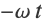about the z-axis.

There is a slight complication in applying the transform to the Hamiltonian, however, due to the time dependence of the unitary transformation. We would like the transformed Hamiltonian to have the properties of a Hamiltonian, i.e., to satisfy the Schrödinger equationin the rotating frame. The fact that the rotating frame is non-inertial, however, means that the Schrödinger equation must be modified by a correction term, analogous to the fictitious force used in classical mechanics to account for the modifications that Newton's laws undergo in an accelerating frame. Alternatively, it is more convenient to define a modified effective Hamiltonian in the rotating frame, so that the Schrödinger equation is the same in all frames. If we define a modified effective Hamiltonian in the rotating frame by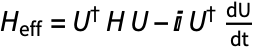, it can be easily shown, starting from the Schrödinger equation in the stationary frame, that this results in the same form for the Schrödinger equation in both the stationary and rotating frames.

 Find the effective Hamiltonian in the rotating frame.
 The function EffectiveHamiltonian can be used to give the same result.

We see that transforming the Hamiltonian to the effective rotating-frame Hamiltonian has two effects: the oscillation frequencies in the off-diagonal elements are shifted by ω, and the energy splitting between the diagonal terms is shifted by the same amount. This second effect is a result of the correction term in the formula for the effective Hamiltonian.

In the optical case that we considered initially, the analogous unitary transformation does not have the same physical interpretation as taking the stationary frame to a rotating frame. Nonetheless, on the strength of the analogy, we continue to refer to the transformed frame as a rotating frame.

In constructing the unitary transformation matrix for the optical case, we can think of shifting the upper state down by a frequency ω in order to simultaneously shift the optical frequency in the resonant coupling term to zero. The unitary transformation is then written as, where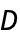is a diagonal matrix with the desired energy shift for each atomic state on the diagonal:

 The unitary matrix for transformation to the interaction frame in the optical case.

The unitary matrix that we find by this method is equivalent to the one used in the spin-1/2 case, up to an overall phase factor. We then find the effective Hamiltonian in the rotating frame:

 Apply EffectiveHamiltonian to the stationary frame and chosen unitary matrix.

The level diagram helps us visualize what we have accomplished. The upper state has been shifted down by the optical frequency ω, so that it now is only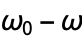above the lower state. The frequencies of the two optical fields have been shifted by the same amount, so that the frequency of one of them is zero (the two ends of the arrow are at the same energy) and the frequency of the other is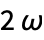. Note, however, that each field has the same detuning from resonance as it did in the stationary frame:below resonance for the near-resonant field, and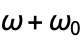below resonance for the off-resonant field.

 Level diagram for the system in the rotating frame, showing both resonant and off-resonant couplings.

Finally, we note that, in a typical atomic system, the far-off-resonant terms (those that oscillate at twice the optical frequency in the effective Hamiltonian) lead to excitations that are so weak that the terms can be neglected to an excellent approximation. This is the rotating-wave approximation.